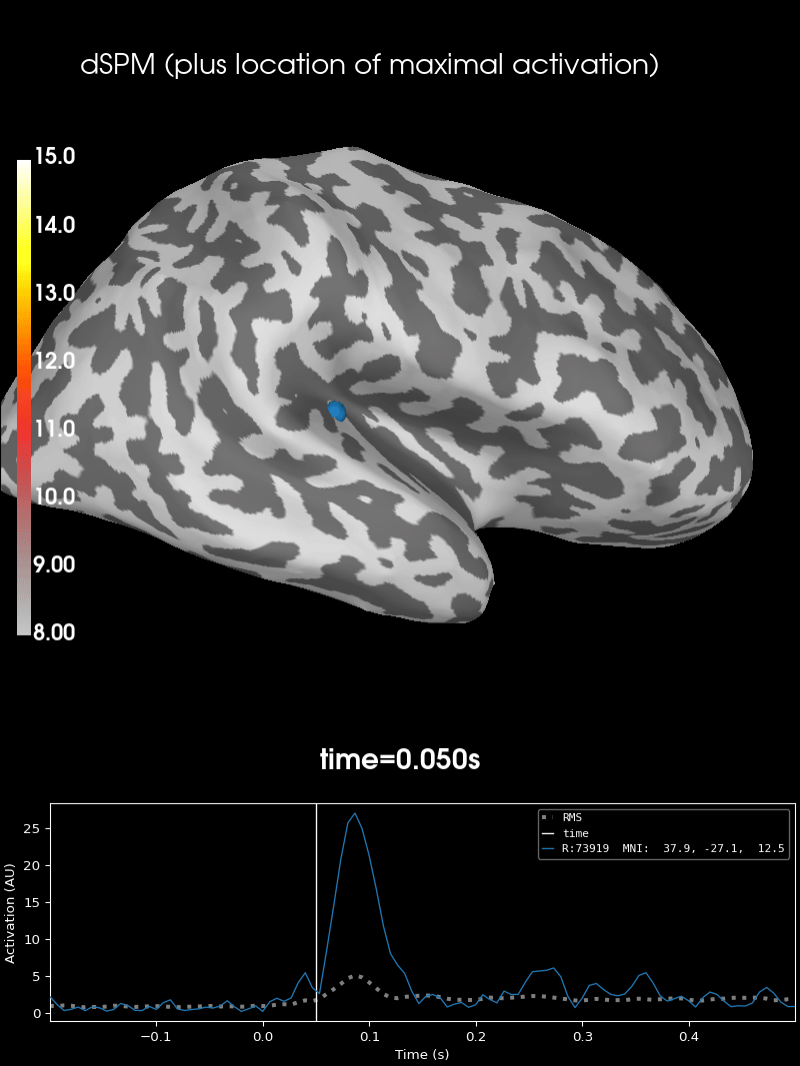# Source localization with MNE, dSPM, sLORETA, and eLORETA#

The aim of this tutorial is to teach you how to compute and apply a linear minimum-norm inverse method on evoked/raw/epochs data.

```import numpy as np
import matplotlib.pyplot as plt

import mne
from mne.datasets import sample
from mne.minimum_norm import make_inverse_operator, apply_inverse
```

Process MEG data

```data_path = sample.data_path()
raw_fname = data_path / "MEG" / "sample" / "sample_audvis_filt-0-40_raw.fif"

events = mne.find_events(raw, stim_channel="STI 014")

event_id = dict(aud_l=1)  # event trigger and conditions
tmin = -0.2  # start of each epoch (200ms before the trigger)
tmax = 0.5  # end of each epoch (500ms after the trigger)
raw.info["bads"] = ["MEG 2443", "EEG 053"]
baseline = (None, 0)  # means from the first instant to t = 0

epochs = mne.Epochs(
raw,
events,
event_id,
tmin,
tmax,
proj=True,
picks=("meg", "eog"),
baseline=baseline,
reject=reject,
)
```
```Opening raw data file /home/circleci/mne_data/MNE-sample-data/MEG/sample/sample_audvis_filt-0-40_raw.fif...
Read a total of 4 projection items:
PCA-v1 (1 x 102)  idle
PCA-v2 (1 x 102)  idle
PCA-v3 (1 x 102)  idle
Average EEG reference (1 x 60)  idle
Range : 6450 ... 48149 =     42.956 ...   320.665 secs
319 events found
Event IDs: [ 1  2  3  4  5 32]
72 matching events found
Setting baseline interval to [-0.19979521315838786, 0.0] s
Applying baseline correction (mode: mean)
Created an SSP operator (subspace dimension = 3)
4 projection items activated
```

## Compute regularized noise covariance#

For more details see Computing a covariance matrix.

```noise_cov = mne.compute_covariance(
epochs, tmax=0.0, method=["shrunk", "empirical"], rank=None, verbose=True
)

fig_cov, fig_spectra = mne.viz.plot_cov(noise_cov, raw.info)
```
••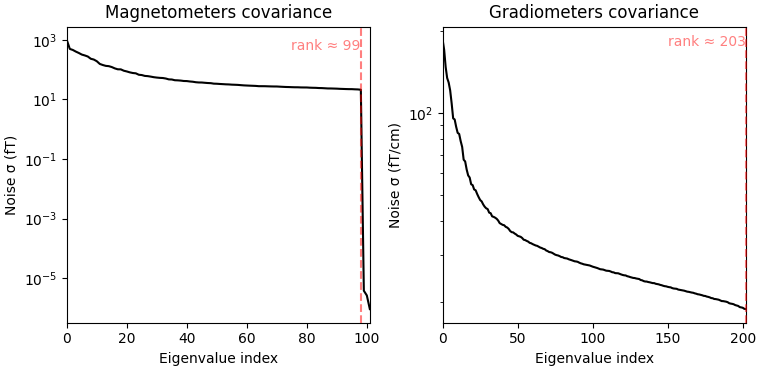```Loading data for 72 events and 106 original time points ...
Rejecting  epoch based on EOG : ['EOG 061']
Rejecting  epoch based on EOG : ['EOG 061']
Rejecting  epoch based on EOG : ['EOG 061']
Rejecting  epoch based on EOG : ['EOG 061']
Rejecting  epoch based on EOG : ['EOG 061']
Rejecting  epoch based on MAG : ['MEG 1711']
Rejecting  epoch based on EOG : ['EOG 061']
Rejecting  epoch based on EOG : ['EOG 061']
Rejecting  epoch based on EOG : ['EOG 061']
Rejecting  epoch based on EOG : ['EOG 061']
Rejecting  epoch based on EOG : ['EOG 061']
Rejecting  epoch based on EOG : ['EOG 061']
Rejecting  epoch based on EOG : ['EOG 061']
Rejecting  epoch based on EOG : ['EOG 061']
Rejecting  epoch based on EOG : ['EOG 061']
Rejecting  epoch based on EOG : ['EOG 061']
Rejecting  epoch based on EOG : ['EOG 061']
Computing rank from data with rank=None
Using tolerance 2.8e-09 (2.2e-16 eps * 305 dim * 4.2e+04  max singular value)
Estimated rank (mag + grad): 302
MEG: rank 302 computed from 305 data channels with 3 projectors
Created an SSP operator (subspace dimension = 3)
Setting small MEG eigenvalues to zero (without PCA)
Reducing data rank from 305 -> 302
Estimating covariance using SHRUNK
Done.
Estimating covariance using EMPIRICAL
Done.
Using cross-validation to select the best estimator.
Number of samples used : 1705
log-likelihood on unseen data (descending order):
shrunk: -1468.506
empirical: -1574.608
selecting best estimator: shrunk
[done]
Computing rank from covariance with rank=None
Using tolerance 2.2e-14 (2.2e-16 eps * 102 dim * 0.98  max singular value)
Estimated rank (mag): 99
MAG: rank 99 computed from 102 data channels with 0 projectors
Computing rank from covariance with rank=None
Using tolerance 1.6e-13 (2.2e-16 eps * 203 dim * 3.5  max singular value)
GRAD: rank 203 computed from 203 data channels with 0 projectors
```

## Compute the evoked response#

Let’s just use the MEG channels for simplicity.

```evoked = epochs.average().pick("meg")
evoked.plot(time_unit="s")
evoked.plot_topomap(times=np.linspace(0.05, 0.15, 5), ch_type="mag")
```
•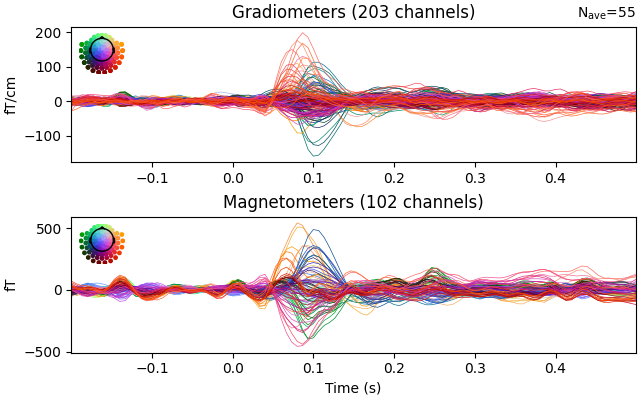•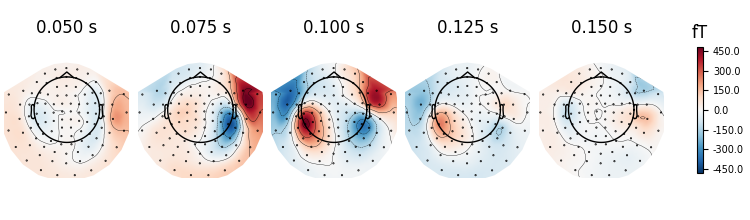```NOTE: pick_channels() is a legacy function. New code should use inst.pick(...).
Removing projector <Projection | Average EEG reference, active : True, n_channels : 60>
```

It’s also a good idea to look at whitened data:

```evoked.plot_white(noise_cov, time_unit="s")
del epochs, raw  # to save memory
```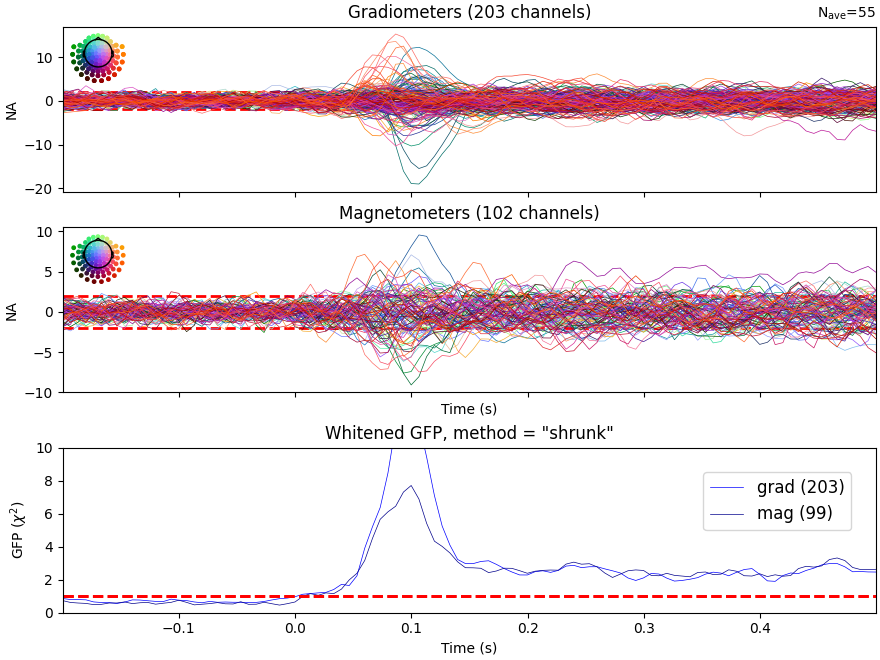```NOTE: pick_types() is a legacy function. New code should use inst.pick(...).
Computing rank from covariance with rank=None
Using tolerance 1.6e-13 (2.2e-16 eps * 203 dim * 3.5  max singular value)
GRAD: rank 203 computed from 203 data channels with 0 projectors
Computing rank from covariance with rank=None
Using tolerance 2.2e-14 (2.2e-16 eps * 102 dim * 0.98  max singular value)
Estimated rank (mag): 99
MAG: rank 99 computed from 102 data channels with 3 projectors
Created an SSP operator (subspace dimension = 3)
Computing rank from covariance with rank={'grad': 203, 'mag': 99, 'meg': 302}
Setting small MEG eigenvalues to zero (without PCA)
Created the whitener using a noise covariance matrix with rank 302 (3 small eigenvalues omitted)
```

## Inverse modeling: MNE/dSPM on evoked and raw data#

Here we first read the forward solution. You will likely need to compute one for your own data – see Head model and forward computation for information on how to do it.

```fname_fwd = data_path / "MEG" / "sample" / "sample_audvis-meg-oct-6-fwd.fif"
```
```Reading forward solution from /home/circleci/mne_data/MNE-sample-data/MEG/sample/sample_audvis-meg-oct-6-fwd.fif...
Computing patch statistics...
[done]
Computing patch statistics...
[done]
Desired named matrix (kind = 3523) not available
Read MEG forward solution (7498 sources, 306 channels, free orientations)
Source spaces transformed to the forward solution coordinate frame
```

Next, we make an MEG inverse operator.

```inverse_operator = make_inverse_operator(
evoked.info, fwd, noise_cov, loose=0.2, depth=0.8
)
del fwd

# You can write it to disk with::
#
#     >>> from mne.minimum_norm import write_inverse_operator
#     >>> write_inverse_operator('sample_audvis-meg-oct-6-inv.fif',
#                                inverse_operator)
```
```Converting forward solution to surface orientation
Average patch normals will be employed in the rotation to the local surface coordinates....
Converting to surface-based source orientations...
[done]
Computing inverse operator with 305 channels.
305 out of 306 channels remain after picking
Selected 305 channels
Creating the depth weighting matrix...
203 planar channels
limit = 7265/7498 = 10.037795
scale = 2.52065e-08 exp = 0.8
Applying loose dipole orientations to surface source spaces: 0.2
Whitening the forward solution.
Created an SSP operator (subspace dimension = 3)
Computing rank from covariance with rank=None
Using tolerance 2.9e-13 (2.2e-16 eps * 305 dim * 4.3  max singular value)
Estimated rank (mag + grad): 302
MEG: rank 302 computed from 305 data channels with 3 projectors
Setting small MEG eigenvalues to zero (without PCA)
Creating the source covariance matrix
Computing SVD of whitened and weighted lead field matrix.
largest singular value = 4.67933
scaling factor to adjust the trace = 9.16538e+18 (nchan = 305 nzero = 3)
```

## Compute inverse solution#

We can use this to compute the inverse solution and obtain source time courses:

```method = "dSPM"
snr = 3.0
lambda2 = 1.0 / snr**2
stc, residual = apply_inverse(
evoked,
inverse_operator,
lambda2,
method=method,
pick_ori=None,
return_residual=True,
verbose=True,
)
```
```Preparing the inverse operator for use...
Scaled noise and source covariance from nave = 1 to nave = 55
Created the regularized inverter
Created an SSP operator (subspace dimension = 3)
Created the whitener using a noise covariance matrix with rank 302 (3 small eigenvalues omitted)
Computing noise-normalization factors (dSPM)...
[done]
Applying inverse operator to "aud_l"...
Picked 305 channels from the data
Computing inverse...
Eigenleads need to be weighted ...
Computing residual...
Explained  65.2% variance
Combining the current components...
dSPM...
[done]
```

## Visualization#

We can look at different dipole activations:

```fig, ax = plt.subplots()
ax.plot(1e3 * stc.times, stc.data[::100, :].T)
ax.set(xlabel="time (ms)", ylabel="%s value" % method)
```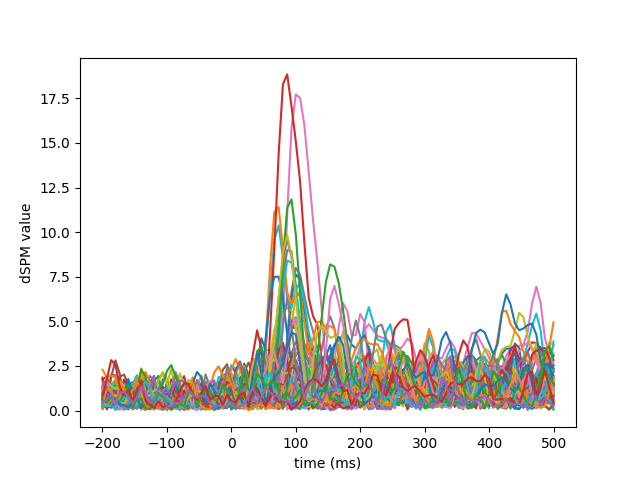Examine the original data and the residual after fitting:

```fig, axes = plt.subplots(2, 1)
evoked.plot(axes=axes)
for ax in axes:
for text in list(ax.texts):
text.remove()
for line in ax.lines:
line.set_color("#98df81")
residual.plot(axes=axes)
```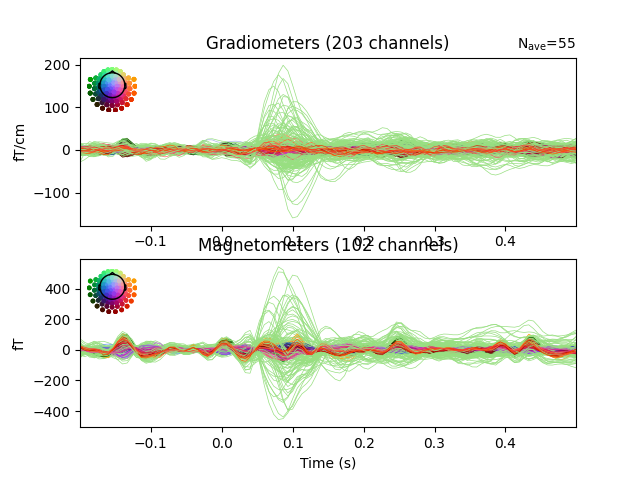Here we use peak getter to move visualization to the time point of the peak and draw a marker at the maximum peak vertex.

```vertno_max, time_max = stc.get_peak(hemi="rh")

subjects_dir = data_path / "subjects"
surfer_kwargs = dict(
hemi="rh",
subjects_dir=subjects_dir,
clim=dict(kind="value", lims=[8, 12, 15]),
views="lateral",
initial_time=time_max,
time_unit="s",
size=(800, 800),
smoothing_steps=10,
)
brain = stc.plot(**surfer_kwargs)
vertno_max,
coords_as_verts=True,
hemi="rh",
color="blue",
scale_factor=0.6,
alpha=0.5,
)